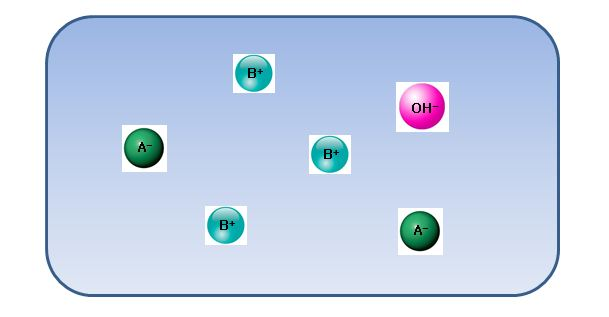# Ionic model

Chemistry Level 2The above is an ionic model for the mixture of strong acid aqueous solution and strong base aqueous solution. Which of the following explanations is correct about this?

a) The mixed solution is perfectly neutral, i.e. its $\text{pH}$ is 7.

b) $\ce{A-}$ ion and $\ce{B+}$ ion are spectator ions.

c) The number of ions in the acid solution was smaller than that of the base solution before mixing.

×